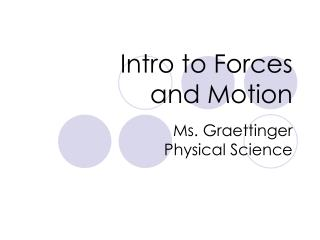# Intro to Forces and Motion - PowerPoint PPT PresentationDownload PresentationIntro to Forces and Motion

Intro to Forces and MotionDownload Presentation## Intro to Forces and Motion

- - - - - - - - - - - - - - - - - - - - - - - - - - - E N D - - - - - - - - - - - - - - - - - - - - - - - - - - -
##### Presentation Transcript

1. Intro to Forces and Motion Ms. Graettinger Physical Science

2. What is a force? • Force is a push or pull on an object or by an object • Force= mass x acceleration • F=ma • A force is that which changes or tends to change the state of rest or motion of a body.

3. Newton’s 3 Laws • 1st : An object in motion stays in motion. An object at rest stays at rest--until an outside force acts upon it • 2nd : F=ma • 3rd : For every action, there is an equal and opposite reaction

4. The Force Equation • F = ma • Force = mass x acceleration • Mass is in kg • Acceleration is in m/s2 • Force is in Newtons (N)

5. Force Equation Example • How much force is needed to accelerate a 70-kg rider and her 200-kg motorcycle at 4 m/s2? • F=ma • F=(70kg + 200kg) x (4 m/s2) • F=1080 N

6. Falling Objects • Any two objects falling from the same distance, released at the same time, will land at the same time • Why is this?

7. Acceleration due to Gravity • Falling objects accelerate at 9.8m/s2 • Therefore, • Weight=m x a • W=m x 9.8m/s2

8. Air Resistance • The force air exerts on a moving object • If there is no force except for gravity, all objects will accelerate at a rate of 9.8 m/s2

9. Air Resistance • This force acts in the opposite direction to that of the objects motion • Air resistance pushes up while gravity pulls down

10. Air Resistance Example

11. Classwork:Everyone must calculate how much they really weigh in Newtons.Conversion factor: 1kg=2.2 lb